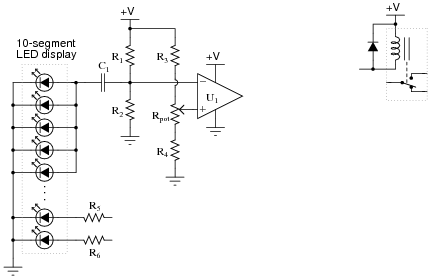# Learning Electronics

Learn to build electronic circuits

# Light-pulse switch

### Question 1:

It is fairly important to use large value resistors (10 MW works well) for resistors R1 and R2, as well as a comparator with high input impedance (a TL08x operational amplifier with JFET inputs is fine). Explain why.
The (internal) Thévenin equivalent resistance for the LED light sensor is quite high, making it sensitive to loading.

Notes:
Nothing to note here.

### Question 2:

Suppose a student builds this circuit, using 10 MW resistors for R1 and R2, and a 22 mF capacitor for C1, and finds that he must frequently adjust the potentiometer to keep the circuit at a reasonable level of sensitivity. A fellow student has encountered and overcome this same problem, and offers to fix the first student's circuit by replacing the 22 mF capacitor with something much smaller: a 0.001 mF (1 nF) capacitor.
Now, the modified circuit responds predictably. No longer must the student frequently adjust the potentiometer to achieve the desired sensitivity. Explain why a change in capacitance made such a difference, and what the problem was in the original circuit (with the much larger capacitor).
Here's a hint: calculate the time constant (t) for the RC network formed by R1, R2, and C1.

Notes:
In order to understand the problem, students must recognize the function of this RC network: to filter out the DC bias voltage created by ambient light falling on the LED array, and to replace that changing bias with a fixed bias voltage equal to [1/2] Vsupply. Once this purpose is grasped, the problem of a slow time constant should be much more apparent.

### Question 3:

Modify the circuit shown to use a 555 monostable timer instead of a J-K flip-flop at the output of the comparator.Also, explain the significance of reversing the comparator's input connections (as compared to the schematic shown on the front page of this worksheet). Why is it better to do this, if we are triggering a monostable 555 timer circuit?
I'll let you and your classmates figure out the answer(s) to this question.

Notes:
Nothing to note here.